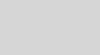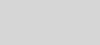# Find the Index Number of a Value in a PowerShell ArrayDr Scripto

Summary: In this blog post, Microsoft Scripting Guy, Ed Wilson, talks about finding the index number of a value in a Windows PowerShell array.

Microsoft Scripting Guy, Ed Wilson, is here. The other day, the Scripting Wife and I were invited to speak with a customer in Charlotte. Our contact, Rafael, is a really cool guy (he is also a SQL Server MVP). We met Rafael at various SQL Saturday events in the southern portion of the United States. It was great to see our friend again, and the group was extremely engaged and asked lots of great questions. One question I received had to do with arrays, namely, “How can I determine the element number of an array to see the data in that array?”

Note: This is the third blog post in a series devoted to working with arrays in Windows PowerShell. In the first post, Learn Simple Ways to Handle Windows PowerShell Arrays, I discussed creating arrays, indexing into arrays, and two techniques for walking through an array. In yesterday’s post, Add, Modify, Verify, and Sort Your PowerShell Array, I discussed adding, modifying, or verifying values in a Windows PowerShell array, and two easy techniques for sorting the array.

# Find the index of value in an array

The first thing I need to do is create an array that contains a number of random numbers. (This is not a requirement to find an index value in an array, but it is a requirement for the demo. Also, being able to create a random array like this is useful when it comes to practicing with arrays.) I like to use the Get-Random cmdlet to do this because it is easier than doing a bunch of typing. Here is the command to create an array that contains 10 random numbers.

\$array = Get-Random -Count 10 -in(1..100)

## Use the forstatement

Now, I use a for statement with a pipeline that begins at zero, a test pipeline that continues until i is 1 less than the length of the array, and an increment pipeline that increases the value of i by 1. Here is the for condition.

for(\$i=0;\$i-le \$array.length-1;\$i++)

The script block that is associated with the for statement uses parameter substitution and the format operator to display the counter (\$i) value and the value that is contained in the specific array element. Here is the script block portion of the for statement.

{“`\$array[{0}] = {1}” -f \$i,\$array[\$i]}

The command to create an array of random numbers and display the array element numbers in addition to the values that are contained in the array element are shown in the following image.## Using the indexofstatic method

The System.Array .NET Framework class contains a number of static methods that are useful. These static methods are shown here (they are all well documented on MSDN).

PS C:\> [array] | gm -s | select name, definition | ft -a

Name            Definition

—-            ———-

BinarySearch    static int BinarySearch(array array, System.Object value), static…

Clear           static System.Void Clear(array array, int index, int length)

ConstrainedCopy static System.Void ConstrainedCopy(array sourceArray, int sourceI…

ConvertAll      static TOutput[] ConvertAll[TInput, TOutput](TInput[] array, Syst…

Copy            static System.Void Copy(array sourceArray, array destinationArray…

CreateInstance  static array CreateInstance(type elementType, int length), static…

Equals          static bool Equals(System.Object objA, System.Object objB)

Exists          static bool Exists[T](T[] array, System.Predicate[T] match)

Find            static T Find[T](T[] array, System.Predicate[T] match)

FindAll         static T[] FindAll[T](T[] array, System.Predicate[T] match)

FindIndex       static int FindIndex[T](T[] array, System.Predicate[T] match), st…

FindLast        static T FindLast[T](T[] array, System.Predicate[T] match)

FindLastIndex   static int FindLastIndex[T](T[] array, System.Predicate[T] match)…

ForEach         static System.Void ForEach[T](T[] array, System.Action[T] action)

IndexOf         static int IndexOf(array array, System.Object value), static int …

LastIndexOf     static int LastIndexOf(array array, System.Object value), static …

ReferenceEquals static bool ReferenceEquals(System.Object objA, System.Object objB)

Resize          static System.Void Resize[T](T[]& array, int newSize)

Reverse         static System.Void Reverse(array array), static System.Void Rever…

Sort            static System.Void Sort(array array), static System.Void Sort(arr…

TrueForAll      static bool TrueForAll[T](T[] array, System.Predicate[T] match)

To use the indexof static method, I provide an array and a value that I want to find. This is shown here.

[array]::indexof(\$array,39)

The command to create an array of 10 random numbers, display the contents of the array, find the index number of one item in the array, and then verify that value is shown in the following image.# Work with one half of the array

It is common to need to work with one half of an array at a time. As it turns out, this is incredibly easy to do—I simply use the range operator. To test this, I first want to create an array with 20 elements in it. To do this, I use the range operator and store the array of numbers in a variable. This command is shown here.

\$array = 1..20

Next, I test to ensure that I have 20 elements in my array. I do this by displaying the content as shown here.

PS C:\> \$array

1

2

<output truncated>

20

Instead of displaying the content of a rather large array, I could use the Count or the Length properties as shown here.

PS C:\> \$array.count

20

PS C:\> \$array.Length

20

I now use the range operator and the GetUpperBound method to display the first half of the array. This command and its associated output are shown here.

PS C:\> \$array[0..(\$array.GetUpperBound(0)/2)]

1

2

3

4

5

6

7

8

9

10

11

Well, the previous command works alright, but the problem seems to be that it displayed one additional element: –element 11. To fix this requires subtracting one from the upper boundary of the array. Here is the revised code and its output.

PS C:\> \$array[0..((\$array.GetUpperBound(0)/2)-1)]

1

2

3

4

5

6

7

8

9

Bummer! That did not work as I had expected. I then decide to examine the value with which I am working. Here is the result.

PS C:\> (\$array.GetUpperBound(0)/2)

9.5

How did I get 9.5 when I have 20 elements in my array? Well, this is because the upper boundary of the array is 19 (remember we start counting at 0). I then decide to “cheat” and convert the value to an integer. The revised command and output are shown here.

PS C:\> \$array[0..([int](\$array.GetUpperBound(0)/2)-1)]

1

2

3

4

5

6

7

8

9

10

With this in mind, I can now access the second half of the array. Here is my command to accomplish that task.

PS C:\> \$array[[int](\$array.GetUpperBound(0)/2)..(\$array.GetUpperBound(0))]

11

12

13

14

15

16

17

18

19

20

That is all there is to finding a value in an array when you do not know the index number. Array Week will continue tomorrow when I will talk about working with arrays of arrays.

I invite you to follow me on Twitter and Facebook. If you have any questions, send email to me at scripter@microsoft.com, or post your questions on the Official Scripting Guys Forum. See you tomorrow. Until then, peace.

Ed Wilson, Microsoft Scripting Guy